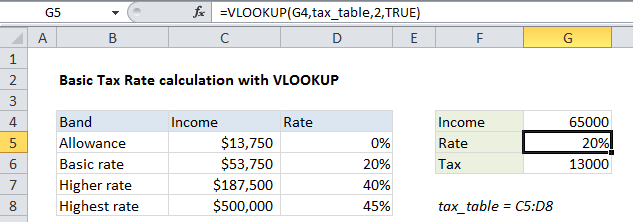## Excel Office

Excel How Tos, Tutorials, Tips & Tricks, Shortcuts

# Basic Tax Rate calculation with VLOOKUP in Excel

This tutorial shows how to calculate Basic Tax Rate calculation with VLOOKUP in Excel using the example below;

## Formula

`=VLOOKUP(amount,tax_table,2,TRUE)`## Explanation

To calculate a tax rate based on a simple tax rate table, you can use the VLOOKUP function. In the example shown, the formula in G5 is:

`=VLOOKUP(G4,tax_table,2,TRUE)`

## How this formula works

Note: This formula depends on a simple tax table with numeric data in the first column, sorted from lowest to highest. The first column in the table represents “lookup values”.

Worked Example:   VLOOKUP with multiple criteria in Excel

The solution requires only the VLOOKUP function:

• The lookup value itself comes from G4
• The table array is the named range tax_table
• The column index number is 2, since the actual tax rates are in the second column
• Finally, the range_lookup argument is set to TRUE, to allow an approximate match
Worked Example:   Multiple chained VLOOKUPs in Excel

With this configuration, VLOOKUP scans the lookup values until it finds a value higher than the value in G4, then VLOOKUP “drops back” to the previous row and returns the tax rate from the second column in the table.

Worked Example:   VLOOKUP with two client rates in Excel

### VLOOKUP matching modes

VLOOKUP has two matching modes: exact match and approximate match, controlled by the forth argument, called range_lookup. The range_lookup argument is optional and defaults to TRUE, but in this case it is set explicitly to TRUE for clarity.